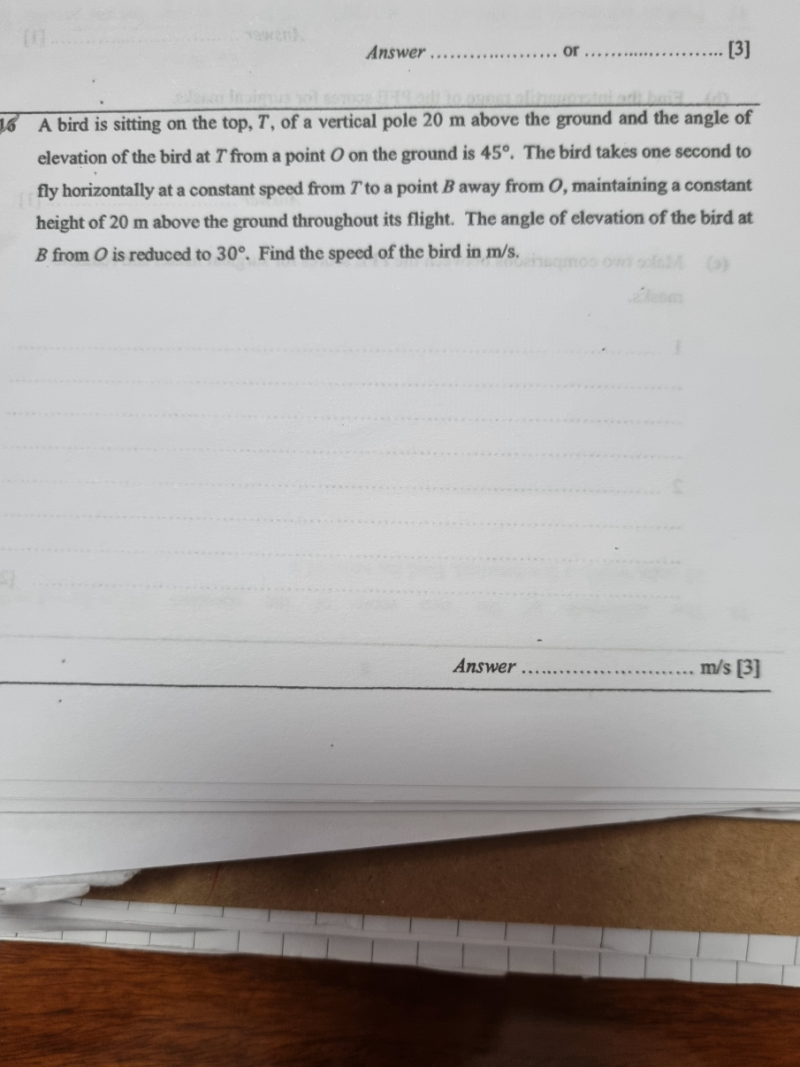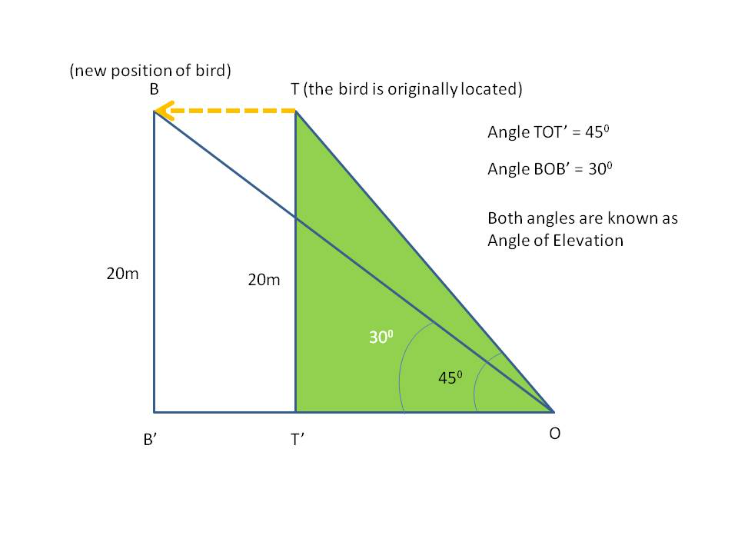# QuestionThank you.20/ OT ‘ = tan 45 = 1

OT ‘ = 20

20 / OB’ = tan 30 =  1 / sqrt(3)

OB’ = 20 sqrt(3)

So distance travelled by bird = OB’ – OT’ = 20 sqrt(3) – 20

Time taken by bird = 1 s

So Speed of bird = Distance / time =  [20 sqrt(3) – 20 ] / 1 = 14.64 m/s  ( to 2 decimal places)

0 Replies 2 Likes ✔Accepted Answer